Published: 30 June 2014

# Gearbox degradation analysis using narrowband interference cancellation under non-stationary conditions

Xinghui Zhang1
Jianshe Kang2
Eric Bechhoefer3
Lei Xiao4
Jianmin Zhao5
1, 2, 5Mechanical Engineering College, Shijiazhuang, 050003, China
3Green Power Monitoring System, LLC, Cornwall, VT, USA
4State Key Lab of Mechanical Transmission, Chongqing University, Chongqing, 400030, China
Corresponding Author:
Xinghui Zhang
Views 152

#### Abstract

Condition-based maintenance (CBM) has become a hot research topic in recent decades. For enhancing the CBM ability, many researchers pay attention to the incipient fault detection and remaining useful life (RUL) prediction. Detecting faults earlier can provide enough lead time to manage the maintenance actions. Besides of this, predicting RUL more accurate can avoid the over maintenance and utilize the components’ life sufficiently. As we know, good degradation features are very important for RUL prediction. However, extracting a monotonous degradation feature is easier for stationary condition than non-stationary condition. In order to address above two issues, this paper uses a new signal processing method named narrowband interference cancellation (NIC) to do degradation analysis. It can extract periodic impulse signals from very noise background which mainly contains narrowband signals. So, the incipient faults can be found easier. The energy ratio calculated based on NIC can be used as degradation feature under non-stationary condition. To verify the effectiveness of NIC based processing, two gearbox run-to-failure tests were conducted. One is under relative stationary condition; the other is under non-stationary condition. The results of these two experiments demonstrate the validity of proposed methods.

## 1. Introduction

Gearboxes are one of the most important components of transmission systems of rotating machineries such as helicopters, vehicles, and wind turbines etc. Effective condition monitoring works of these gearboxes can save much maintenance costs and avoid many catastrophes. In recent decades, incipient fault detection, fault diagnosis and prognosis have become the hot research contents of condition-based maintenance (CBM) . For some enterprises, capital equipments’ failures will lead to great economical loss and has big security risks. Therefore, they prefer to detect the faults earlier and replace the fault components timely. For implementing CBM effectively, the incipient fault detection is very important to support enough lead time for planning the maintenance actions.

For vibration signals of gearboxes, impulsive signals produced by incipient faults are usually immersed in noises. Therefore, many methods are developed to enhance the impulsive signals or to suppress the noises. Wang and Jiang  proposed an adaptive wavelet threshold de-noising method to enhance the fault feature and used correlation dimension as the degradation indicator. Sun et al.  proposed a multi-wavelet de-noising technique with the data-driven block threshold. The optimal block length and threshold at different decomposition levels can be selected using minimum Stein’s unbiased risk estimate. Yan and Lu  proposed an improved Hilbert-Huang transform (HHT) to detect the incipient fault. In this improved HHT, wavelet analysis was used to remove the iterative errors in the sifting process and a new stopping criterion based on correlation analysis was developed. Abad et al.  used discrete wavelet transform and artificial neural network to detect the gear faults based on acoustic signals. However, useful signals also will be suppressed when de-nosing. Except of this, the gear signal will be noise when we only want to detect the bearing faults. Many de-noising methods will not work in this case. So, many researchers pay attention to series methods which mainly filter the useless signals out or enhance the weak impulsive signal produced by faults. Wang and Wong used autoregressive (AR) model to remove the regular toothmesh pattern for enhancing the impulsive signal produced by gear faults . Endo and Randall  proposed the use of minimum entropy deconvolution (MED) technique to enhance the ability of the existing AR model based filtering technique to detect localized faults in gears. Under this fundamental, some other revised AR models are also developed to detect the gear faults under constant load [8, 9]. Then, Yang and Makis further developed an ARX model based gear fault detection and localization under varying load conditions. This method considers load as additional information and tests validated it can be used in real situations . Recently, in order to overcome the deficiency of MED, McDonald et al.  proposed an improved novel deconvolution norm, Correlated Kurtosis, which takes advantage of the periodicity of the faults and requires no AR model stage prior to deconvolution. For signals of gear faults, it usually contains many narrowband signals (gear vibration signals of normal mesh motion) and broad noise which mask the desired periodic impulsive signals produced by gear faults. If the narrowband signals can be filtered out, the impulsive signals will be manifest. According to this character, Bechhoefer  used a new method named Narrowband Interference Cancellation (NIC) to enhance the gear faults detection. This method can enhance the periodic impulsive signal. Therefore, it can be used to detect incipient faults.

The remainder of this article can be organized as follows. Section 2 introduces the NIC theory and the energy ratio calculation. Section 3 describes the two RTF experiments and detail test process. Section 4 concentrates on the enhanced fault detection based on NIC. The degradation indicator extraction based on NIC under non-stationary condition and comparison work with the AR model and sideband index are shown in Section 5. Section 6 has a discussion and future work outlook. Section 7 concludes the paper.

## 2. Theoretical background of narrowband interference cancellation

For gear faults, they will produce impulse signals with relative characteristic frequencies. So the vibration signals collected from machines contain gear mesh, shaft rotation, bearing vibration and random noise. For the signals produced by gear and shaft, they are narrowband. But the impulse signals generated by gear faults are in a wideband. Usually, gear fault signals are very weak compared to the gear mesh tones and shaft rotation. So, if we can filter out these narrowband signals, the signals of gear faults can be detected easier. A machine system can be modeled as:

1
$x\left(n\right)=s\left(n\right)+y\left(n\right)+v\left(n\right),$

where $s\left(n\right)$ is the signal of interest, $y\left(n\right)$ is the signal associated with gear mesh, shafts rotation, e.g. interference, $v\left(n\right)$ is random noise.

The interference signal is usually large compared to the signal of interest. It is necessary to remove the interfering signal $y\left(n\right)$ from $x\left(n\right)$ while preserving the signal of interest $s\left(n\right)$. Since the measured signal $x\left(n\right)$ and the interference signal $y\left(n\right)$ are correlated, one can estimate the interference using an optimal linear estimator:

2
$\stackrel{^}{y}\left(n\right)={\mathbf{c}}_{0}^{H}x\left(n-D\right),$
3
$\mathbf{R}{\mathbf{c}}_{0}=\mathbf{d},$
4
$\mathbf{R}=E\left\{\mathbf{x}\left(n-D\right){\mathbf{x}}^{H}\left(n-D\right)\right\},$
5
$\mathbf{d}=E\left\{\mathbf{x}\left(n-D\right){y}^{*}\left(n\right)\right\},$

where $D$ is an integer delay operator. If $D=$1, then Eq. (2) is the least square forward linear predictor. If $\stackrel{^}{y}\left(n\right)=y\left(n\right)$, the output of the filter is $x\left(n\right)-\stackrel{^}{y}\left(n\right)=s\left(n\right)+v\left(n\right)$. This means we can completely remove the interference and only the desire signal and noise remains. In practice, signal $y\left(n\right)$ is not available. To overcome this obstacle, we can use a minimum means square error $D$-step forward linear predictor, such that:

6
${e}^{f}\left(n\right)=x\left(n\right)+{\mathbf{a}}^{H}\mathbf{x}\left(n-D\right),$
7
$\mathbf{R}\mathbf{a}=-{\mathbf{r}}^{f},$

where:

8
${\mathbf{r}}^{f}=E\left\{\mathbf{x}\left(n-D\right){x}^{*}\left(n\right)\right\}.$

For this modeling, the components of the observed signal have the following properties:

• The signal of interest $s\left(n\right)$, the interference signal $y\left(n\right)$, and the noise signal $v\left(n\right)$ are mutually uncorrelated.

• The noise signal $v\left(n\right)$ is white.

• The signal of interest $s\left(n\right)$ is wideband and has a short correlation length (e.g. it’s impulsive).

• The interference signal $y\left(n\right)$ has a long correlation length: its autocorrelation length takes significant values over the range $0\le |l|\le M$, for $M>D$.

In practice, the second and third properties mean that the desired signal and the noise are approximately uncorrelated after a certain small lag. These are precisely the properties exploited by the canceller to separate the narrowband interference from the desired signal and the background noise.

According to the first assumption, we have:

9
$E\left\{x\left(n-k\right){y}^{*}\left(n\right)\right\}=E\left\{y\left(n-k\right){y}^{*}\left(n\right)\right\}={r}_{y}\left(k\right),$
10
${r}_{x}\left(l\right)={r}_{s}\left(l\right)+{r}_{y}\left(l\right)+{r}_{v}\left(l\right).$

If the second and third modeling assumptions hold true, we have:

11
${r}_{x}\left(l\right)={r}_{y}\left(l\right)\text{for}l\ne 0,1,\dots ,D-1.$

The exclusion of the lags for $l\ne 0,1,\dots ,D-1$ in $\mathbf{r}$ and $\mathbf{d}$ is critical, and we have arranged for that by forcing the filter and the predictor to form their estimates using the “delayed” data vector $\mathbf{x}\left(n-D\right)$. From Eqs. (5), (8), and (11), we conclude that $\mathbf{d}={\mathbf{r}}^{f}$ and therefore ${\mathbf{c}}_{0}={\mathbf{a}}_{0}$. Thus, the optimum narrowband interference estimator ${\mathbf{c}}_{0}$ is equal to the $D$-step linear predictor ${\mathbf{a}}_{0}$, which can be determined exclusively from the input signal $x\left(n\right)$. Then, the signal with interference removed is:

12
$x\left(n\right)-\stackrel{^}{y}\left(n\right)=x\left(n\right)-{\text{a}}_{\text{0}}^{H}x\left(n-D\right)=ef\left(n\right),$

which is identical to the $D$-step forward prediction error. This leads to the linear prediction NIC shown in Fig. 1. For a full description of the analysis, we can see .

Fig. 1Block diagram of linear prediction NBI canceller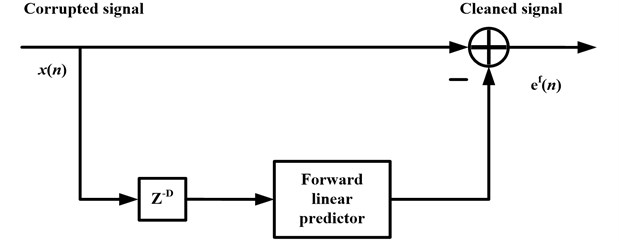From the above analysis, we know the $s\left(n\right)$ is the periodic impulsive signal produced by gear faults and the $y\left(n\right)$ denotes the normal vibration signal except the faults. The purpose of the NIC is to filter the $y\left(n\right)$ out from the signal $x\left(n\right)$. This means the NIC can separate the deterministic part and the residual part. So, we can use $y\left(n\right)$ to denote the deterministic part and the $x\left(n\right)-y\left(n\right)$ to denote the residual part. Therefore, the energy ratio based on NIC (ER-NIC) can be depicted as:

13
$\text{Energy ratio}=\frac{{\sum }_{n=1}^{N}y{\left(n\right)}^{2}}{{\sum }_{n=1}^{N}{\left(x\left(n\right)-y\left(n\right)\right)}^{2}}.$

## 3. Experiment setup

In order to validate the effectiveness of proposed method, there are two gearbox RTF data sets can be used. One was operated under relative stationary conditions. The other was operated under non-stationary conditions. These data sets were collected in the full life gearbox degradation processes. Vibration signals collected at each inspection point is relative stationary. This means the speed and load of gearbox will not vary in a very short time. For experiment 1, the speed and load had a very small fluctuation around a certain value in the whole degradation process. However, for experiment 2, the speed and load were varying with a great degree.

Fig. 2a) Test-rig of gearbox, b) Gearbox structure and sensor location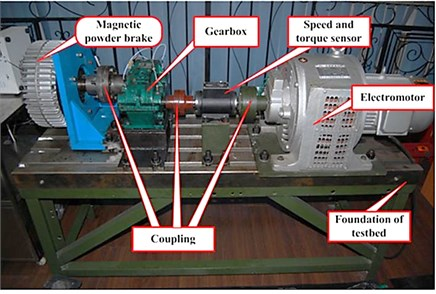a)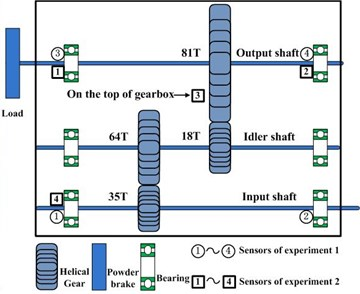b)

The experiment system used in this paper can be shown in Fig. 2(a). This system contains a gearbox, a 4 kW three phase asynchronous motor for driving the gearbox, and a magnetic powder brake for loading. The motor is an electromagnetic speed-adjustable motor which allows the tested gear to operate under various speeds. As depicted in Fig. 2(b), the gearbox has three shafts, which are mounted to the gearbox housing by rolling element bearings. Gear on low speed (LS) shaft has 81 teeth and meshes with gear on the right intermediate speed (IS) shaft which has 18 teeth. Gear on left IS shaft has 64 teeth and meshes with gear on the high speed (HS) shaft which has 35 teeth. These teeth information are very important for us to know the mesh frequencies. The data acquisition system contains acceleration transducers, PXI-1031 mainframe, PXI-4472B data acquisition cards, and LabVIEW software. The type of transducers is 3065B4 of Dytran Company. There are four transducers which are mounted in different places on gearbox. In order to acquire the speed and torque information, a speed and torque transducer is installed in the input shaft as depicted in Fig. 2(a). For this transducer, one shaft revolution will produce 60 impulses.

Fig. 3a) Fault propagation over time of experiment 1, b) Fault propagation over time of experiment 2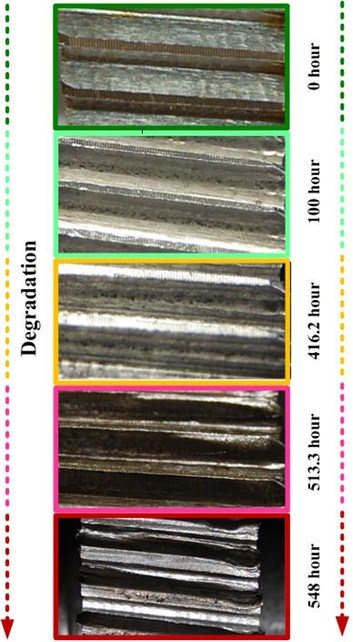a)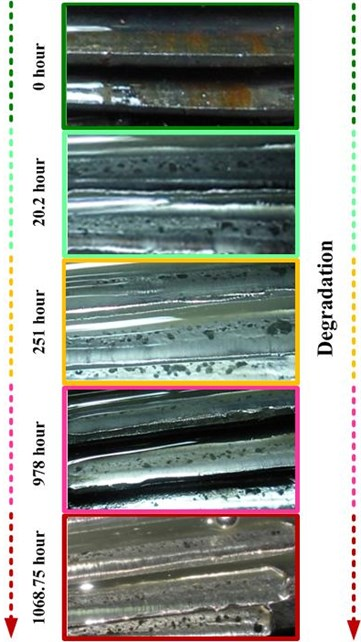b)

For experiment 1, the transducers’ locations are denoted using circle symbols in Fig. 2(b). The rotation speed of input shaft is about 1,200 rpm and the torque is about 15 Nm. The operation conditions are relative stationary because of the small fluctuation. In this experiment process, the vibration signals were acquired every ten minutes and last 12 seconds. The sampling frequency is 20 KHz. When the experiment system operated 548 hours, the gear of LS shaft had many broken teeth and this experiment was ended. The whole degradation process can be depicted as follows. When a normal gear operates some time, some pitting faults will appear on the gear face. With the time elapse, these pitting faults will extend to the spalling and lead to broken teeth finally. The degradation process of LS shaft gear can be depicted in Fig. 3(a).

For experiment 2, the transducers’ locations are denoted using square symbols in Fig. 2(b). The rotation speed and torque were controlled to vary with time. The rotation speed was varied in range 200-1200 rpm and the torque was varied in range 0-60 Nm. The operation conditions are non-stationary because of the large fluctuation. The vibration signals were acquired every 5 minutes and last 2 seconds. Similar to the experiment 1, the sampling frequency is 20 KHz. When the experiment system operated 1068.75 hours, the gear of the right IS shaft had severe wear of many teeth and the experiment was ended. The degradation process of this gearbox is very similar to the experiment 1. The degradation process of the IS shaft gear can be depicted in Fig. 3(b).

In order to show the rotation speed and torque variation of these two experiments. They can be calculated through the speed and torque signal acquired in the experiment process. After calculation, they can be depicted in Fig. 4 and Fig. 5.

Fig. 4a) Speed variation of experiment 1, b) Torque variation of experiment 1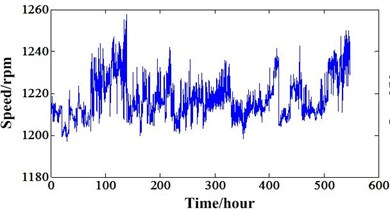a)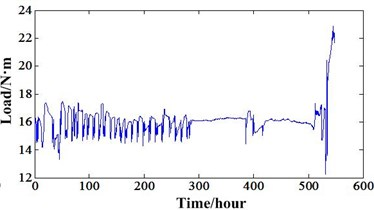b)

Fig. 5a) Speed variation of experiment 2, b) Torque variation of experiment 2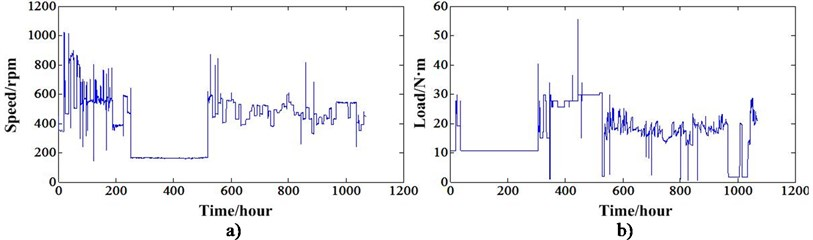## 4. Enhanced fault detection based on NIC

Take experiment 1 for example, in order to demonstrate the effectiveness of enhanced fault detection ability based on NIC, the data collected near the failure is selected as the analysis object. This data was collected at time point 3,241 (interval between two inspections is ten minutes). The data of sensor 4 which is near the gear of LS shaft is used. Because the rotation speed has small fluctuation near the 1200 rpm, the signal was resampled using time synchronous technology to remove the variation of rotation speed first. The HS shaft is the synchronous shaft. Then, it is processed by NIC to extract the impulsive signal produced by gear faults while suppressing the narrowband signal produced by no fault components. In this analysis, the parameters $D$ and $M$ of NIC are selected as 1 and 4096. The FFT spectrum of resampled signal can be seen in Fig. 6(a). After NIC processing, the FFT spectrum can be seen in Fig. 6(b).

In the Fig. 6, GMF denotes gear mesh frequency. IS-LS denotes the gear mesh of IS shaft and LS shaft. HS-IS denotes the gear mesh of HS shaft and IS shaft. The Fig. 6(b) showed that the narrowband signals which mainly the mesh frequency of HS-IS and its harmonics were suppressed. In order to further validate the proposed method, the detail spectrum of mesh frequency of IS-LS and its harmonics can be illustrated as Figs. 7-9.

Fig. 6a) FFT spectrum of resampled signal, b) FFT spectrum after NIC processing of resampled signal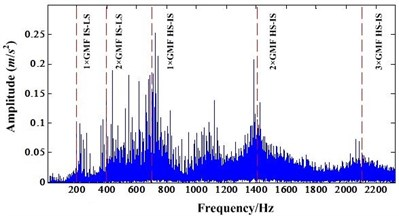a)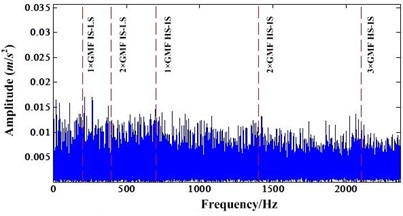b)

Fig. 7a) Detail spectrum of first order IS-LS GMF before NIC processing, b) Detail spectrum of first order IS-LS GMF after NIC processing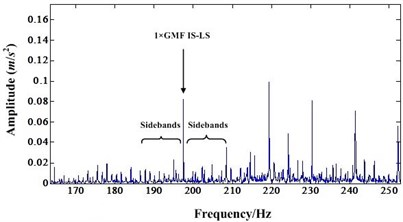a)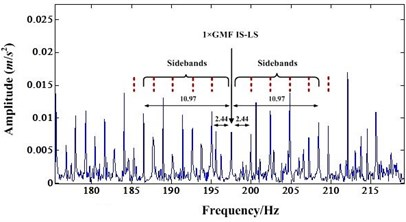b)

Fig. 8a) Detail spectrum of second order IS-LS GMF before NIC processing, b) Detail spectrum of second order IS-LS GMF after NIC processing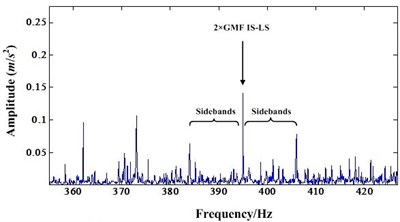a)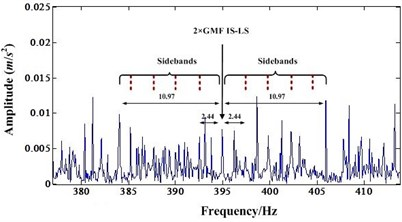b)

Fig. 9a) Detail spectrum of third order IS-LS GMF before NIC processing; b) Detail spectrum of third order IS-LS GMF after NIC processing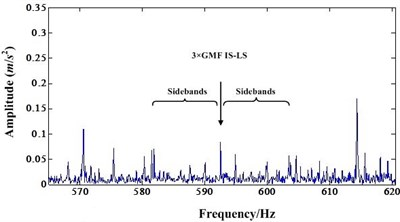a)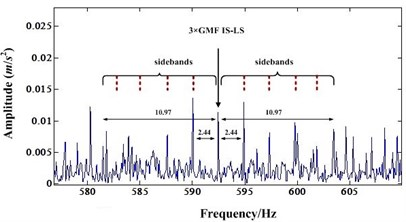b)

Fig. 7(a) is the spectrum around the mesh frequency with a frequency range from 170 Hz to 250 Hz (the first order mesh frequency of IS-LS is 197.5 Hz). It shows that there is a IS shaft rotation modulation sideband occurring around the mesh frequency component. There is also a first order LS shaft rotation modulation sideband around the mesh frequency component. However, the amplitude of this first order sideband is very small compared to the amplitude of mesh frequency component. Fig. 8(a) and Fig. 9(a) are very similar to Fig. 7(a). In these figures, the sidebands are not dominant.

After NIC processing, the impulse signals are extracted while the narrowband signals are suppressed. Fig. 7(b), Fig. 8(b) and Fig. 9(b) are the detail spectrum after NIC processing. A careful study of these figures indicates that the LS shaft rotation sidebands become dominant. In particular, the mesh frequency components are almost immersed by the sidebands. These Figures shows that some sidebands have higher amplitude than the mesh frequency component. This test indicates that NIC technology can enhance the impulsive signal produced by gear faults which leads to fault detection easier. Therefore, when some incipient faults emerged for the gear, NIC can detect these faults easier than other methods.

## 5. Degradation analysis based on NIC

Many traditional statistical features developed especially for gear fault diagnosis only can track the gear degradation under relative stationary operating conditions. These features are ER (Energy ratio), FM0, FM4, FM4*, NA4, NB4, M6A and M8A etc . Reference  had a detail analysis of part of these features. This paper only shows the results of FM4 and FM4* of the two RTF tests. They can be depicted in Fig. 10 and Fig. 11.

Fig. 10a) FM4 value of experiment 1, b) FM4* value of experiment 1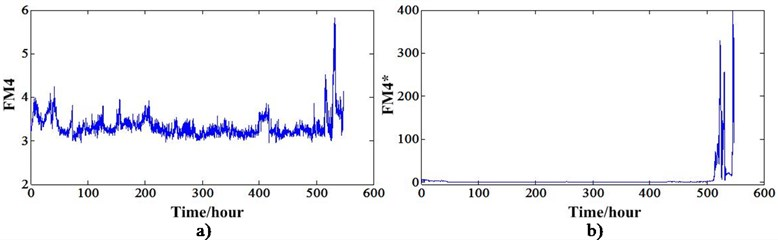Fig. 11a) FM4 value of experiment 2, b) FM4* value of experiment 2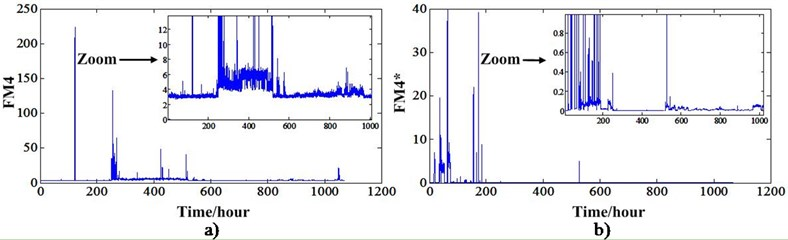From these two figures, we can see that FM4 and FM4* can track the degradation of experiment 1 but cannot track the degradation of experiment 2. This further demonstrates these two features can only reflect the degradation under stationary conditions. When the operation conditions fluctuate in a great extent, these features also will fluctuate with the varying speed and load. This type features cannot be used to do the RUL prediction. For experiment 1, Fig. 10 shows that the FM4 and FM4* have an increase trend only after the 500 hours. This means even if the RUL prediction methods have a good performance, the RUL is not enough for planning the maintenance actions. In order to overcome this problem, Zhang et al.  developed a new sideband index enhanced by the NIC. This indicator has an increase trend at about 400 hours. This means there will have more hours to plan the maintenance actions and lower the risks of breakdown. This new degradation indicator can be depicted in Fig. 12(a). In order to do a comparison with non-stationary conditions indicator, this sideband index also extracted for the experiment 2 as depicted in Fig. 12(b). From this figure, we know this indicator also can work only under the relative stationary conditions. Otherwise, this indicator will have a great fluctuation with the varying conditions. This new sideband index is extracted based on the time synchronous and NIC processing. The HS shaft is the synchronous shaft and data of sensor 4 is used because it outperforms other sensor data.

Fig. 12a) Sideband index based NIC of experiment 1, b) Sideband index based NIC of experiment 2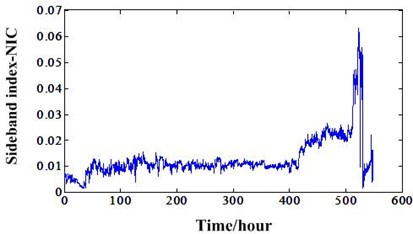a)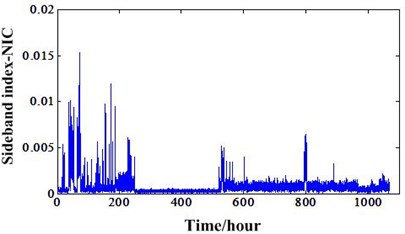b)

Fig. 13a) ER-NIC value of experiment 1, b) ER-NIC value of experiment 2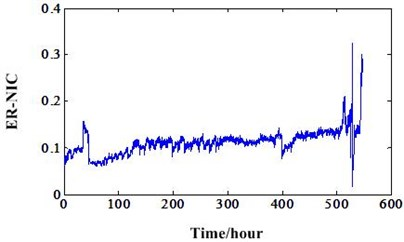a)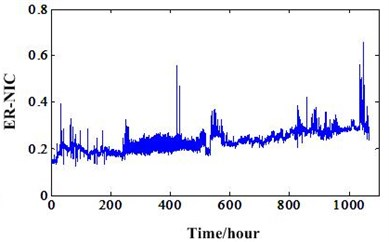b)

Then, the new energy ratio value mentioned in Section 2 should be investigated based on these two different RTF experiments. Many traditional indicators developed for gear fault diagnosis and prognosis need to process the signal using time synchronous averaging (TSA) algorithm. However, for this new energy ratio, it not needs the TSA processing which will make it useless. This new energy ratio values of the two experiments based on NIC can be depicted in Fig. 13. It shows that this new feature can track the gear degradation better than other traditional statistical features under the non-stationary conditions. In addition, we can also compare this indicator with the sideband index value based on NIC of experiment 1. This comparison can be illustrated in Fig. 14. It shows ER-NIC degradation indicator is better than the sideband index value based on NIC. From the overall degradation process view, the ER-NIC indicator has better trend which are suitable for the RUL prediction than sideband index value based on NIC. As shown in ellipse of the figure, the sideband index value is decreased at the end of the gearbox life because of the operation conditions’ influence. However, the ER-NIC has not been influenced by the varying operation conditions and has a good trend. So, we can conclude the ER-NIC can be used as a good degradation feature for RUL prediction regardless of the operation conditions. Fig. 15 is the energy ratio based on AR (ER-AR) model. Compared to the Fig. 13(b), we can see ER-NIC has a good performance than ER-AR. Features FM4, FM4*, ER-NIC of experiment 1 was extracted from the data of sensor 2. For experiment 2, these features and ER-AR were extracted from the data of sensor 1. Because the energy ratios extracted from these sensors are the best. So, in order to keep consistence, others features were extracted from the data of same sensors.

Fig. 14Comparison of ER-NIC and Sideband index based NIC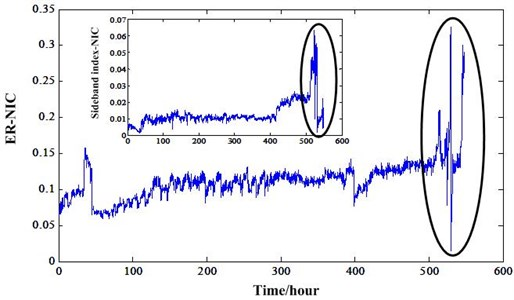Fig. 15AR model based energy ratio of experiment 2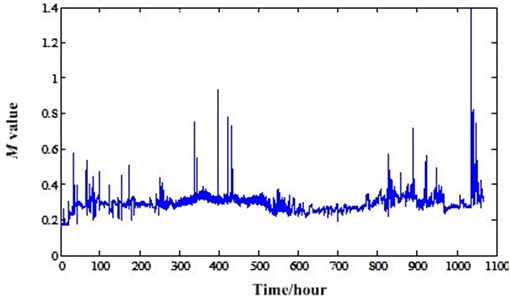There are two parameters in NIC calculation. They are $D$ and $M$ which can be found in Section 2. Different parameters will lead to different results and effectiveness. So, we need to optimize these parameters enabling the NIC has better perfoemance. Usually, the value of $D$ is one according to the experience which will be a least square linear predictor. The value of $M$ is always the power of two such as 16, 32, 64 etc. So, we only need to optimize the value of $M$ in this paper. We consider NIC has a better performane when the residual siganl has a high Kurtosis value. So, we can use Kurtosis value as an indicator to optimize the value of $M$. For the overall degradation process of long RTF experiments, the values of $M$ in different inspection points will be different. So, we need to give $M$ a value which can make most of the residual signals of inspection points more impulsive. The optimum $M$ values of whole degradation processes of the two experiments can be calculated and illustrated in Fig. 16 and Fig. 17. It shows that the optimum values of $M$ should be 25 and 26 for the two experiments. In the optimization process, we only set the $M$ value in a power range [4, 10], because too big $M$ value will lead to the computer out of memory.

Fig. 16Optimum M value variation of degradation process of experiment 1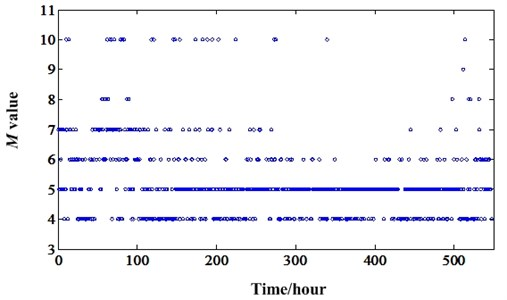Fig. 17Optimum M value variation of degradation process of experiment 2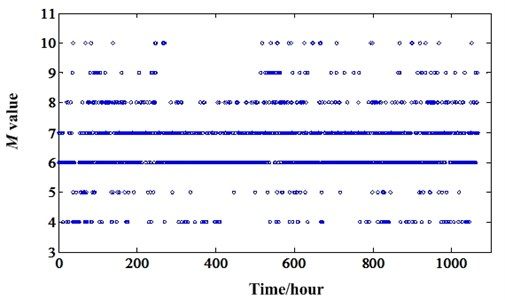## 6. Discussion and future work

From the above data analysis based on NIC presented in Section 4 and 5, we can conclude the NIC can be used to do the incipient fault detection and can extract robust feature for RUL prediction no matter what operation conditions the gearbox faces. However, there are some issues which need to be discussed and do deep research in future work.

1) In the process of ER-NIC feature extraction, it stated the ER-NIC extracted from the time synchrounous signals will do not work. However, the ER-NIC extracted directly from the original signals can have a good degradation trend under varying operating conditions. The reason of this phenomenon shoud be further investigated in future.

2) Except the AR and NIC methods, we need to develop other methods which can separate the deterministic signal from the residual signal. Then, many reliable degradation features can be extracted to facilitate the RUL predictions.

3) For gearbox, if only exist gear faults or bearing faults, the degradation process can be tracked using the proposed method. However, this case only exist in the laboratory. Bearing and gear faults usually exsit at the same time. In most cases, the gear faults can be detect easier than bearing faults. So, how to track the bearing degradation using proposed method when both bearing and gear faults exist need to be researched deeply in future.

4) The ER-NIC can as a good degradation feature mainly because it is not influenced by the varying oprerating conditions. In engineering real, varying operating conditions are a very ordinary phenomenon. So, the NIC can enhance the fault detection ability and can extract a good degradation feature has two reasons. One is it can filter out the fault unrelated signals out. So, the signals produced by faults are enhanced. The other is it indeed separate the vibration signals to two parts (fault signals and non-fault signals). The energies of these two parts of signals always vary with the speed and load. However, the energy ratio of these two parts can remove the condition variation effect. Therefore, it is robust to do degradation analysis under non-stationary conditions.

5) Sometimes, real equipments have complex structures than gearbox experiment system used in this paper. Take vehicles for example, they always have gearbox, engine, and other necessary transimission components. All these components will have great vibrations when it operates. Especially for the gearbox used in vehicle, it has many gears, shafts and bearings. It contains parallel transimission and planetary transimissions. In the condition monitoring work, there will be big noise which will has a bad influence to proposed method in this paper. So, we can set more sensors on the gearbox which can alleviate the energy lost because of the long transimission path. Shorter path between the fault and the sensor will lead to better performance of proposed method.

## 7. Conclusions

In this paper, a new incipient fault detection and degradation feature extraction method under varying operating conditions has been developed. This is an adaptive signal processing method called narrowband interference cancellation. It can filter the narrowband signals out which are mainly the vibration signals of normal gear mesh and shaft rotation. Then, the residual signals are mainly the periodic impulsive signals induced by the gear or bearing faults. This process indeed enhances the fault detection. So, usually when the gearbox has some incipient faults, this method will work well to detect the faults than other methods. In order to address the degradation feature extraction dilemma under non-stationary operating conditions, the energy ratio of deterministic and residual part signals which are separated by NIC is developed. Before extract the degradation feature, the parameter $M$ of NIC is optimized according to the Kurtosis criterion. The proposed methods are verified using two RTF gearbox experiments. At the end, some works which need to be researched deeply in future has been discussed.

02 January 2014
Accepted
06 June 2014
Published
30 June 2014
Keywords